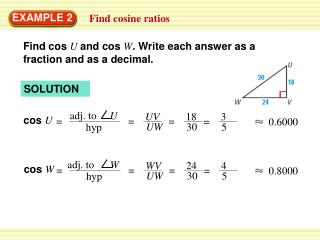DownloadDownload PresentationEXAMPLE 2

# EXAMPLE 2

Download Presentation## EXAMPLE 2

- - - - - - - - - - - - - - - - - - - - - - - - - - - E N D - - - - - - - - - - - - - - - - - - - - - - - - - - -
##### Presentation Transcript

1. adj. to U adj. to W = = hyp hyp WV 3 UV 24 18 4 = = = = = = 0.6000 0.8000 5 30 5 30 UW UW EXAMPLE 2 Find cosine ratios Find cos U and cos W. Write each answer as a fraction and as a decimal. SOLUTION cos U cos W

2. You want to string cable to make a dog run from two corners of a building, as shown in the diagram. Write and solve a proportion using a trigonometric ratio to approximate the length of cable you will need. EXAMPLE 3 Use a trigonometric ratio to find a hypotenuse DOG RUN

3. sin 35o = opp hyp 11 sin 35o = x xsin 35o = 11 x = x 11. 11. sin35o 0.5736 ANSWER x 19.2 You will need a little more than 19 feet of cable. EXAMPLE 3 Use a trigonometric ratio to find a hypotenuse SOLUTION Write ratio for sine of 35o. Substitute. Multiply each side by x. Divide each side by tan. 35o Use a calculator to find tan. 35o Simplify.

4. adj. to R adj. to S = = hyp hyp 12 4 RT ST 9 3 = = = = = = 0.8 0.6 5 SR 15 5 15 SR for Examples 2, and 3 GUIDED PRACTICE In Exercises 3 and 4, find cos Rand cos S. Write each answer as a decimal. Round to four decimal places, if necessary. SOLUTION Find SR, use the Pythagorean Theorem ST2 + TR2 = SR2 122 + 92 = SR2 15 = SR cos R cos S

5. adj. to S adj. to R = = hyp hyp RT ST 30 16 = = = = 0.4706 0.8824 SR 34 34 SR for Examples 2, and 3 GUIDED PRACTICE In Exercises 3 and 4, find cos Rand cos S. Write each answer as a decimal. Round to four decimal places, if necessary. SOLUTION Find SR, use the Pythagorean Theorem ST2 + TR2 = SR2 162 + 302 = SR2 34 = SR cos R cos S

6. adj hyp x 19.2 cos35o = cos 35o = = x 19.2cos35o x = 15.7 for Examples 2, and 3 GUIDED PRACTICE In Example 3, use the cosine ratio to find the length of the other leg of the triangle formed. 5. SOLUTION Write ratio for cosine of 35o. Substitute. multiply each side by 19.2 Simplify.